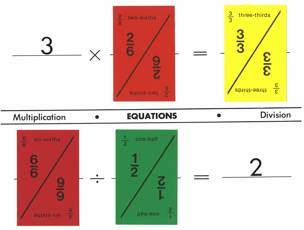Home

EQUATIONS MATS

Use the Addition and Subtraction mat and one of the 10 Multiplication/Division mats to form equations. Select 10 arbitrary Fraction Bars or Fraction Bars Playing Cards and try to place them on the 10 blanks of the two mats to from equations (One example for two mats is shown below). If necessary take one or more bars (cards) to form the 10 equations with as few bars (cards) as possible.